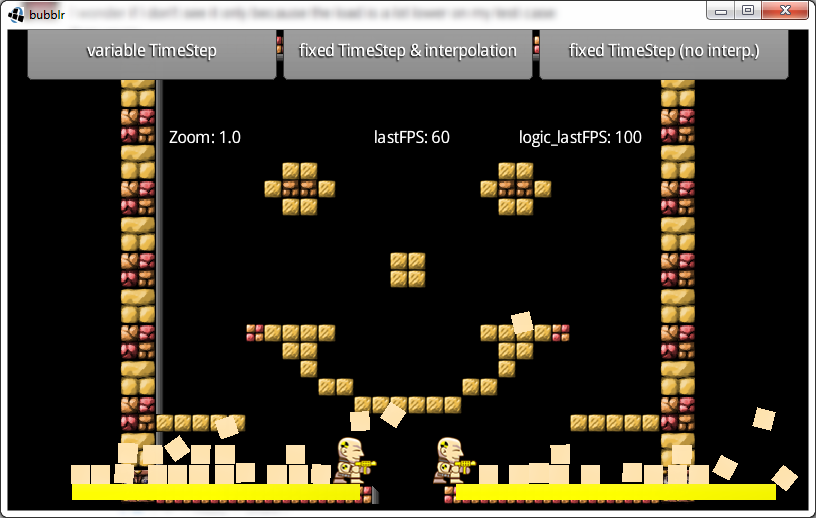Fix your Timestep is a fantastic article by Glenn Fiedler that explains the different ways to tick a physics simulation. Show some self-respect, go read it and check some of his other articles on physics and network code. Some might be 8 years old but still totally relevant.

Essentially, it covers different approaches to pick a delta value to pass through to the physics engine along their strengths and weaknesses. The topic is complex but has an undeniable impact on games' behaviour.

I thought it would be a good idea to show how it translates to a Libgdx application using the Box2D physics engine. Note that it also applies to Bullet, changes would be minimal.

### Simple but wrong approaches

It starts with a fix time step of 1/60th of a second, which makes for a stable simulation but fails terribly when the game runs at less than 60fps, a very common situation in mobile devices.

The logical upgrade is to pass exactly how much time has passed since the last frame. Obviously, not every frame takes the same, which means the simulation could be unstable. Moreover, it will vary across devices as they can feature a wide range of specifications. This is definitely not the way to go.

Everything is lost!

### Fixed timestep

If only we had the best of both worlds! A fixed timestep and a mechanism that caters for the render loop being too slow. As the article explains, the physics will always be stepped by a fixed amount, let's say 1/60s. Some machines may be too fast and render at 120fps, so we can run the physics twice. However, others might be too slow and render at 30fps, in which case we'd only step the physics one every two frames.

What happens when the game runs at 50fps?

We need an `accumulator` where we can put the time spent by the renderer. Then we step the physics by a fixed amount until they sync as best as possible. There might be still be some time left, but we can save it for the next frame.

``````public class SionGame extends ApplicationListener {
private World world;
private double accumulator;
private double currentTime;
private float step = 1.0f / 60.0f;

public void render() {
double newTime = TimeUtils.millis() / 1000.0;
double frameTime = Math.min(newTime - currentTime, 0.25);
float deltaTime = (float)frameTime;

currentTime = newTime;

while (accumulator &amp;gt;= step) {
world.step(step, velIterations, posIterations);
accumulator -= step;
}
}``````

### Fixed timestep with interpolation

The `accumulator <= step` condition in the `while` loop means we can still have some time left in the accumulator but not enough to step the physics at 1/60s. This results in some visual stuttering, as the physics can get slightly out of sync for a while. Glenn Fiedler's suggests we interpolate our entities' transforms between the previous and current physics state based on how much time left is in the accumulator.

``````public class SionGame extends ApplicationListener {
private World world;
private double accumulator;
private double currentTime;
private float step = 1.0f / 60.0f;

public void render() {
double newTime = TimeUtils.millis() / 1000.0;
double frameTime = Math.min(newTime - currentTime, 0.25);
float deltaTime = (float)frameTime;

currentTime = newTime;

while (accumulator &amp;gt;= step) {
world.step(step, velIterations, posIterations);
accumulator -= step;
entityManager.update();
}

entityManager.interpolate(accumulator / step);
}``````

I entirely made `EntityManager` up, it simply should maintain a collection of your game entities. We assume `entityManager.update()` iterates over the list of game entities and updates their position and rotation according to the current physics state. On the other hand, `entityManager.interpolate()` should do something like the following.

``````public void interpolate(float alpha) {
for (Entity entity : entities) {
Transform transform = entity.getBody().getTransform();
Vector2 bodyPosition = transform.getPosition();
Vector2 position = entity.getPosition();
float angle = entity.getAngle();
float bodyAngle = transform.getRotation();

position.x = bodyPosition.x * alpha + position.x * (1.0f - alpha);
position.y = bodyPosition.y * alpha + position.x * (1.0f - alpha);
entity.setAngle(bodyAngle * alpha + angle * (1.0f - alpha));
}
}``````

Although it's been simplified for the purpose of this text, the following snippet comes from SionCore.

### A working sample

Libgdx community memeber, Just4phil, pointed out in the comments the advantages of using a fixed timestep with interpolation. He kindly provided a working sample that lets you switch between all approaches explained in this article. Not only that, he also linked to the source, which is really awesome. Kudos.Again, read the original article if you haven't done so already.